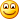# Inserting/Updating Record on related entities using LINQ

It is a very common scenario that you need to insert rows in related tables using LINQ. Assume you have two tables as below,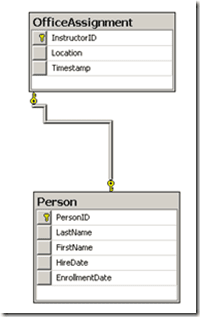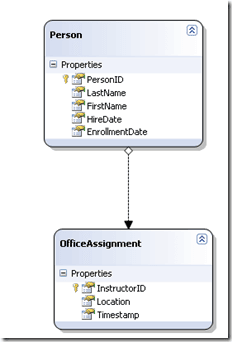Insert a Person with no Office Assignment
If you have requirement to insert a Person without any Office Assignment then it is quiet straight forward task like below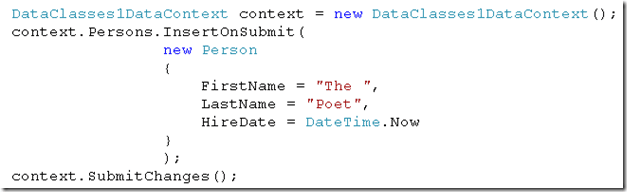If you need to insert Office Assignment while inserting Person, you can create instance of office assignment and insert along with Person like below,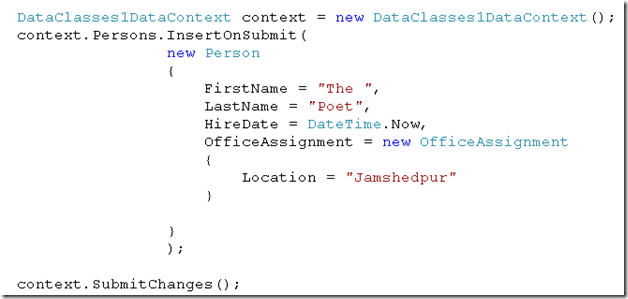Now I have created function to perform both Insert and update operation. If you provide person Id as 0 this function will insert a new row else it will update existing row.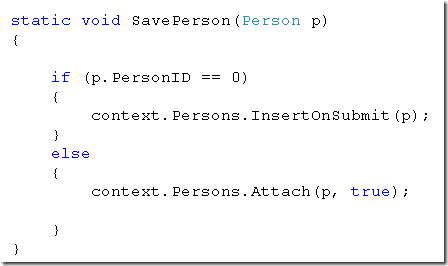When I tried to call above function to update Person with ID 1, I encountered with below exception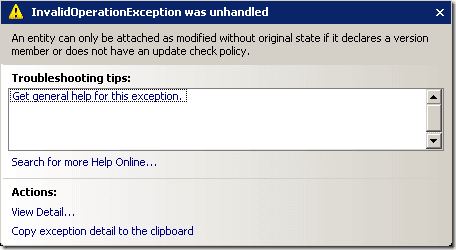To get rid of above exception, you need to call attach with original entity as well. We need to pass modified entity as well as original entity to make a call.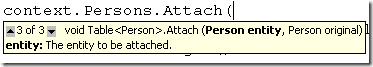So I created a function as below,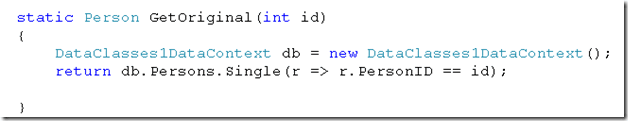And while attaching you need to call above function as below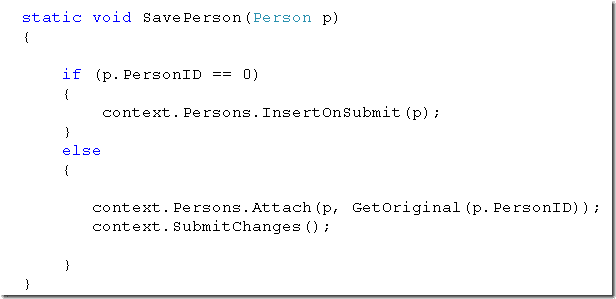Full source code is as below,
 `01` `using` `System;`
 `02` `using` `System.Collections.Generic;`
 `03` `using` `System.Linq;`
 `04` `using` `System.Text;`
 `05`
 `06` `namespace` `Relatedtable`
 `07` `{`
 `08` `    ``class` `Program`
 `09` `    ``{`
 `10`
 `11` `        ``static` `DataClasses1DataContext context;`
 `12` `        ``static` `void` `Main(``string``[] args)`
 `13` `        ``{`
 `14`
 `15` `            ``context = ``new` `DataClasses1DataContext();`
 `16` `            ``context.Log = Console.Out;`
 `17` `            ``context.Persons.InsertOnSubmit(`
 `18` `                            ``new` `Person`
 `19` `                            ``{`
 `20` `                                ``FirstName = ``"The "``,`
 `21` `                                ``LastName = ``"Poet"``,`
 `22` `                                ``HireDate = DateTime.Now,`
 `23` `                                ``OfficeAssignment = ``new` `OfficeAssignment`
 `24` `                                ``{`
 `25` `                                    ``Location = ``"Jamshedpur"`
 `26` `                                ``}`
 `27`
 `28` `                            ``}`
 `29` `                            ``);`
 `30`
 `31` `            ``context.SubmitChanges();`
 `32` `            ``Console.ReadKey(``true``);`
 `33`
 `34` `            ``//Person p = new Person {FirstName ="xuz", LastName = "abdefg" };`
 `35` `            ``//p.OfficeAssignment = new OfficeAssignment { Location = "Mumbai" };`
 `36` `            ``//p.OfficeAssignment = new OfficeAssignment { Location = "Delhi" };`
 `37`
 `38` `            ``//p.`
 `39` `            ``//context.SubmitChanges();`
 `40`
 `41` `            ``Person p = ``new` `Person { PersonID = 1, FirstName = ``"Dhananjay "` `,LastName=``"Kumar"``};`
 `42` `            ``SavePerson(p);`
 `43`
 `44` `            ``var result = from r ``in` `context.Persons select r;`
 `45` `            ``foreach` `(var r ``in` `result)`
 `46` `            ``{`
 `47` `                ``Console.WriteLine(r.FirstName);`
 `48` `            ``}`
 `49` `            ``Console.ReadKey(``true``);`
 `50`
 `51` `            ``var res1 = from r ``in` `context.OfficeAssignments select r;`
 `52` `            ``foreach` `(var a ``in` `res1)`
 `53` `            ``{`
 `54` `                ``Console.WriteLine(a.Location+ a.Person.PersonID);`
 `55` `            ``}`
 `56`
 `57` `            ``Console.ReadKey(``true``);`
 `58`
 `59` `        ``}`
 `60`
 `61` `        ``//static string GetConnectionString(string serverName)`
 `62` `        ``//{`
 `63`
 `64` `        ``//    System.Data.SqlClient.SqlConnectionStringBuilder builder =`
 `65` `        ``//                   new System.Data.SqlClient.SqlConnectionStringBuilder();`
 `66` `        ``//    builder["Data Source"] = devServerName;`
 `67` `        ``//    builder["integrated Security"] = true;`
 `68` `        ``//    builder["Initial Catalog"] = "Sample2";`
 `69` `        ``//    Console.WriteLine(builder.ConnectionString);`
 `70` `        ``//    Console.ReadKey(true);`
 `71` `        ``//    return builder.ConnectionString;`
 `72`
 `73` `        ``//}`
 `74`
 `75` `        ``static` `void` `SavePerson(Person p)`
 `76` `        ``{`
 `77`
 `78` `            ``if` `(p.PersonID == 0)`
 `79` `            ``{`
 `80` `                ``context.Persons.InsertOnSubmit(p);`
 `81` `            ``}`
 `82` `            ``else`
 `83` `            ``{`
 `84`
 `85` `               ``context.Persons.Attach(p, GetOriginal(p.PersonID));`
 `86` `               ``context.SubmitChanges();`
 `87`
 `88` `            ``}`
 `89` `        ``}`
 `90`
 `91` `        ``static` `Person GetOriginal(``int` `id)`
 `92` `        ``{`
 `93` `            ``DataClasses1DataContext db = ``new` `DataClasses1DataContext();`
 `94` `            ``return` `db.Persons.Single(r => r.PersonID == id);`
 `95`
 `96` `        ``}`
 `97` `    ``}`
 `98` `}`
I hope this post was useful. Thanks for reading

Posted by